# XMM-Newton Science Analysis System: User Guide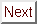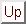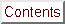Next: 4.12.3 Source detection example Up: 4.12.2 Example of EPIC product generation: images, spectra & light curves Previous: 4.12.2.3 Generation of spectra using efluxer

#### 4.12.2.4 Extraction of a X-ray corrected light curve for a point-like source

As an example case, the extraction of a light curve from a pn event list (PN.evt) will be considered. A similar recipe applies for a MOS event list.

1. Set up your SAS environment (see § 2.3.1)

2. Extract an image (in sky coordinates in this example; extraction in detector - DET[XY] - coordinates is possible as well)

      evselect table=PN.evt:EVENTS imagebinning=binSize \
imageset=PNimage.fits withimageset=yes \
xcolumn=X ycolumn=Y ximagebinsize=80 yimagebinsize=80


3. Display the image

imgdisplay withimagefile=true imagefile=PNimage.fits


4. Select the region, from which the light curve shall be accumulated, using the Region/Circle in ds9 (see figure 30).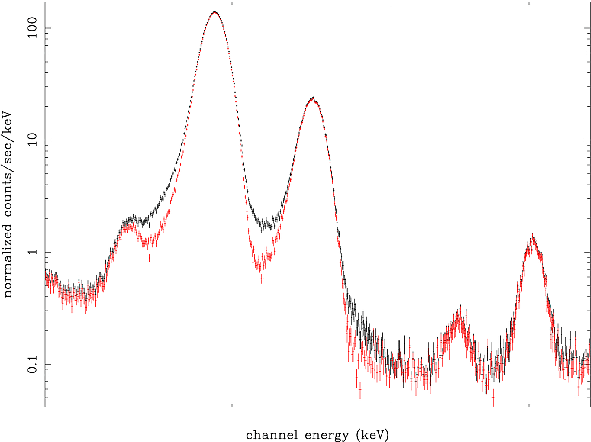5. Double-click with the cursor on the defined region. A window pops up, showing the properties of the region (figure 31). Write down the coordinates of the Center (25910.5, 25870.5) and the Radius (400).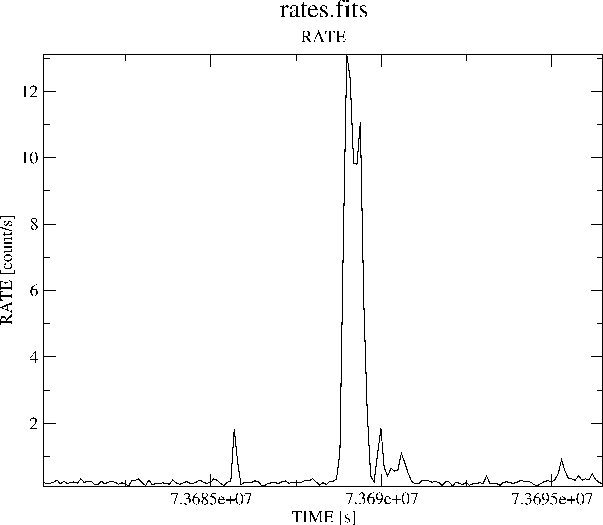Units of sky coordinates (X,Y) are 0.05 arcsec, hence the radius in our example is 20 arcsec.

6. Be aware: if you are interested in very short time periods, such as they appear in pulsars of cataclysmic variables, you have to perform a barycentric correction. This means that the arrival time of a photon is shifted as is it would have been detected at the barycenter of the solar system (the center of mass) instead at the position of the satellite. In this way, the data are comparable. The SAS task barycen performs this correction. It is advisable first to copy the event list since the TIME column of the event list is directly overwritten by the barycentric corrected times

cp PN.evt PN_evlist.fit

barycen table=PN_evlist.fit


7. Now you can extract a source+background light curve, using all the selection expressions defined so far. In the example, the binsize is 100 seconds. Please take into account that operating with non-synchronous time series can introduce artifacts when they are added or subtracted by programmes such as the ftools lcmath. From SAS v8.0 onwards, there is no need to do so, since by default the start time is set to the beginning of the exposure. You can override this by using the parameter timemin and timemax.
      evselect table=PN.evt energycolumn=PI \
expression='#XMMEA_EP&&(PATTERN<=4)&& \
((X,Y) IN circle(25910.5,25870.5,400))&&(PI in [200:10000])' \
withrateset=yes rateset="light_curve.fits" timebinsize=100 \
maketimecolumn=yes makeratecolumn=yes timemin=126991800 \
timemax=130000000


The parameter makeratecolumn=yes produces a light curve in count rates (with errors). Otherwise the light curve is produced in counts (with errors).

8. Repeat step 4. to 6. above to determine the region, from which the background light curve is to be extracted. It will be assumed in what follows that the extraction region correspond to an annulus, centered in (25910.5,25870.5) and with inner and outer radii 1000 and 2000 pixels, respectively.

9. Extract a background light curve, using all the selection expressions defined so far, and the same binsize (100 seconds) and energy range as for the source+background light curve
      evselect table=PN.evt energycolumn=PI \
expression='#XMMEA_EP&&(PATTERN<=4)&& \
((X,Y) IN annulus(25910.5,25870.5,1000,2000)) \
&& (PI in [200:10000])' \
withrateset=yes rateset="light_curve_background.fits" \
timebinsize=100 \
maketimecolumn=yes makeratecolumn=yes \
timemin=126991800 timemax=130000000


The light curves are OGIP-complaint, and therefore analyzable with standard XRONOS-like (Xronos) LHEASOFT packages.

10. However, light curves obtained in such a way should be corrected to account for a number of effects which can have an impact in the detection efficiency, like vignetting, bad pixels, PSF variation and quantum efficiency, as well as to account for time dependent corrections within a exposure, like dead time and GTIs. Since all these corrections can differ between source and background light curves, the background subtraction has to be done accordingly. The SAS task epiclccorr performs all of these corrections at once. It requires as input both light curves (which are used to establish the binning of the final corrected background subtracted light curve) and the event file. A simple command line call:
       epiclccorr srctslist=PN_lightcurve_raw.FIT \
eventlist=PN_evlist.FIT \
outset=PN_lccorr.fit \
bkgtslist=PN1_lc_bck.FIT \
withbkgset=yes \
applyabsolutecorrections=yes


11. Plot the resulting light curves, eg.
       dsplot table=PN_lccorr.fit withx=yes x=TIME withy=yes y=RATE


This command will launch the window shown in figure 32.Next: 4.12.3 Source detection example Up: 4.12.2 Example of EPIC product generation: images, spectra & light curves Previous: 4.12.2.3 Generation of spectra using efluxer
European Space Agency - XMM-Newton Science Operations Centre